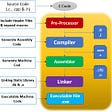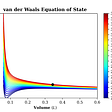# Numpy Vectorization Speed Test with Random Walk Example

`import numpy as npimport random`

# Random Walk

A random walk is a path that has no clear direction but is determined by a series of random decisions, each of which is left entirely to chance. For example, a pollen grain floating on a drop of water moves across the surface of the water because it’s constantly pushed around by water molecules. Molecular motion in a water drop is random, so the path a pollen grain traces on the surface is a random walk. 

The random walk hypothesis is a financial theory stating that stock market prices evolve according to a random walk (so price changes are random) and thus cannot be predicted. It is consistent with the efficient-market hypothesis. Burton G. Malkiel, an economics professor at Princeton University and writer of ‘A Random Walk Down Wall Street’, performed a test where his students were given a hypothetical stock that was initially worth fifty dollars. The closing stock price for each day was determined by a coin flip. If the result was heads, the price would close a half point higher, but if the result was tails, it would close a half point lower. Thus, each time, the price had a fifty-fifty chance of closing higher or lower than the previous day. Cycles or trends were determined from the tests. Malkiel then took the results in a chart and graph form to a chartist, a person who “seeks to predict future movements by seeking to interpret past patterns on the assumption that ‘history tends to repeat itself’”.Random walk hypothesis test by increasing or decreasing the value of a fictitious stock based on the odd/even value of the decimals of pi. The chart resembles a stock chart.

The chartist told Malkiel that they needed to immediately buy the stock. Since the coin flips were random, the fictitious stock had no overall trend. Malkiel argued that this indicates that the market and stocks could be just as random as flipping a coin. 

We can calculate the time execution of a Python statement or expression using the timeit module.
The code was written on Jupyter notebook. So, %timeit magic command was used as below. In the case of a python script, please refer to timeit documentation.
The code is based on blog by Nicolas P. Rougier .

## Object-Oriented Approach

In the Object-Oriented Approach, it is easier to read and comprehend our intended purpose. The code generates ‘-1’ or ‘1’ integer value randomly for each loop and adds the value to the position variable which is then appended to the walk list.

`class RandomWalker:    def __init__(self):        self.position = 0    def walk(self,n):        self.position = 0        for i in range(n):            yield self.position            self.position += 2*random.randint(0,1) - 1walker = RandomWalker()%timeit walk = [position for position in walker.walk(100000)]10 loops, best of 3: 163 ms per loop`

Here, we can see that the object-oriented approach took 163 ms per loop.

## Procedural Approach

For such a simple problem, we can probably save the class definition and concentrate only on the walk method that computes successive positions after each random step.

`def random_walk(n):    position = 0    walk = [position]    for i in range(n):        position += 2*random.randint(0,1)-1        walk.append(position)    return walk%timeit random_walk(100000)10 loops, best of 3: 151 ms per loop`

In the procedural approach, we gained 7% computation time per loop.

## Vectorized Approach

Vectorized Python itertools Approach

Python’s itertools module standardizes a core set of fast, memory-efficient tools that are useful by themselves or in combination. Together, they form an “iterator algebra” making it possible to construct specialized tools succinctly and efficiently in pure Python.

Here, we first generate all the steps at once and use the accumulate function to compute all the positions. We get rid of the loop and this makes things faster.

`from itertools import accumulatedef random_walk_faster(n=100000):    steps = random.choices([-1,+1], k=n)    return +list(accumulate(steps))%timeit walk = random_walk_faster(100000)10 loops, best of 3: 29.3 ms per loop`

In python’s inbuilt vectorization approach we gained 80% computation time per loop as compared to procedural approach.

Vectorized Numpy Approach

Fast and versatile, the NumPy vectorization, indexing, and broadcasting concepts are the de-facto standards of array computing today. Here we use numpy’s cumsum function which returns the cumulative sum of the elements along a given axis.

Numpy is a very powerful library and you can make wonders with it but, most of the time, this comes at the price of readability. So, it’s better to comment the code for future understanding.

`def random_walk_fastest(n=100000):    steps = np.random.choice([-1,+1], n)    return np.cumsum(steps)%timeit walk = random_walk_fastest(100000)1000 loops, best of 3: 1.14 ms per loop`

Using Numpy we gained over 95% computational speed per loop over python’s built-in vectorization approach.

--

--

--

## More from Subash Basnet

I am a Computer Engineer graduated from Kathmandu University, Nepal. The existence is a program, we are here to add our part of code. Website: sbasnet.com.np

Love podcasts or audiobooks? Learn on the go with our new app.

## Useful code snippets for Xamarin development## Lao-Top foundation2## Beta Updates — March 2021## Pushing static type checking in Python one step further## What happens when you type gcc main.c## Why You Should Be a Volunteer On a Software Engineering Conference?## The Ambassador Edge Stack & Ambassador API Gateway 1.6 Now Available## Subash Basnet

I am a Computer Engineer graduated from Kathmandu University, Nepal. The existence is a program, we are here to add our part of code. Website: sbasnet.com.np

## LET’S SEE WHAT’S IN LOOPING!!## Your First Websocket Client in R## Van der Waals Equation of State in Python